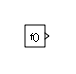# Function-Call Generator

Provide function-call events to control execution of a subsystem or model

• Library:
• Simulink / Ports & Subsystems

•## Description

The Function-Call Generator block provides function-call events that execute a function-call subsystem or function-call model at the rate you specify with the Sample time parameter. To iteratively execute each function-call block multiple times at each time step, use the Number of iterations parameter.

To execute multiple function-call subsystems or models in a specified order, use the Function-Call Generator block with a Function-Call Split block. For an example, see Function-Call Split.

## Ports

### Output

expand all

Sends function-call events to the function-call input port of a function-call subsystem or function-call model, that is, a subsystem or model containing a Trigger block with Trigger type set to `function-call`.

## Parameters

expand all

Specify the time interval between function-call events to a function-call subsystem or model. If the actual calling rate for the subsystem or model differs from the time interval this parameter specifies, Simulink® displays an error.

By default, the block uses a time interval of `1`. To set a different interval, enter a valid sample time based on the table in Types of Sample Time, or specify `-1` to inherit the time interval from the model.

#### Programmatic Use

 Block Parameter: `sample_time` Type: character vector Values: `'1'` | scalar expression | vector expression | `'-1'` Default: `'1'`

The value of this parameter can be a vector, where each element of the vector specifies a number of times to execute a function-call subsystem or model. If a vector is specified, then the block outputs a vector of function-call events, which can be split into individual function-call events using a Demux block and used to drive multiple function-call subsystems or models.

Suppose that you specify Number of iterations to be `[3 2]` and connect the output of this block to a Demux block, which is connected to the control ports of two function-call subsystems. In this case, the first function-call subsystem executes three times at each time step, and the second function-call subsystem executes two times at each time step.

If, however, you connect the output of this block to a single function-call subsystem or model, the total number of times that the function-call subsystem or model executes per time step is equal to the sum of the vector element values. So in the example above, specifying Number of iterations to be `[3 2]` and connecting the output of this block to a single function-call subsystem results in the function-call subsystem executing five times at each time step.

#### Settings

`1`

Provide function-call once during each time step.

integer

Signed or unsigned integer number. Provide the specified number of function calls at each time step.

#### Programmatic Use

 Block Parameter: `numberOfIterations` Type: character vector Values: `'1'` | `''````''``` Default: `'1'`

When this parameter is not selected, the output of this block is expanded into a vector, if necessary, to drive the block(s) to which it is connected. For example, if Number of iterations is set to `2` and Disallow wide output is not selected, and the output of this block is connected to a Demux block, which is in turn connected to three separate function-call subsystems, then each function-call subsystem receives two function-call events at each time step. A similar result occurs if the output of this block is connected to a Stateflow® chart with multiple events. If Disallow wide output is selected in these situations, Simulink produces an error.

Select this parameter to prevent implicit expansion of this block's output signal.

Clear this parameter to allow implicit expansion of this block's output signal when appropriate.

#### Programmatic Use

 Block Parameter: `DisallowWideOutput` Type: character vector Values: `'off'` | `'on'` Default: `'off'`

## Block Characteristics

 Data Types Direct Feedthrough `no` Multidimensional Signals `no` Variable-Size Signals `no` Zero-Crossing Detection `no`